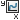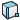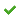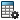Plotting the Momentum of a Part

You can plot the momentum of a part as it moves relative to another part or relative to the assembly origin.

1. From a Motion Analysis study, click Results and Plots(MotionManager toolbar).
2. In the PropertyManager, under Result, for Category, select Momentum/Energy/Power.
3. For Subcategory, select from the following:
• Translational Momentum to calculate the momentum due to the translational or linear motion of a part.
• Angular Momentum to calculate the momentum due to the rotational motion of a part.
4. For Select result component, select an option:
• Magnitude to resolve the magnitude in the direction of the result vector with respect to global coordinates.
• X Component, Y Component, or Z Component to calculate the magnitude of the result in the selected direction.
Optionally, you can select a reference partto plot the result relative to the coordinates of that part.
5. For features, select a part face to calculate the result for the motion of the part.
6. Select the Plot Results options you require, and click.
The result appears in the MotionManager tree.
7. Click Calculate(MotionManager toolbar).